# AP SSC 10th Class Physics Important Questions Chapter 12 Electromagnetism

AP State Board Syllabus AP SSC 10th Class Physical Science Important Questions Chapter 12 Electromagnetism.

## AP State Syllabus SSC 10th Class Physics Important Questions 12th Electromagnetism

### 10th Class Physics 12th Lesson Electromagnetism 1 Mark Important Questions and Answers

Question 1.
Draw the diagram showing the magnetic field lines of bar mannet. (TS June 2016)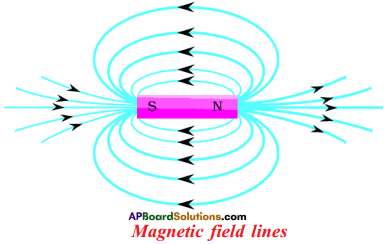Question 2.
Correct the diagram according to Lenz law and draw it again. (TS March 2017)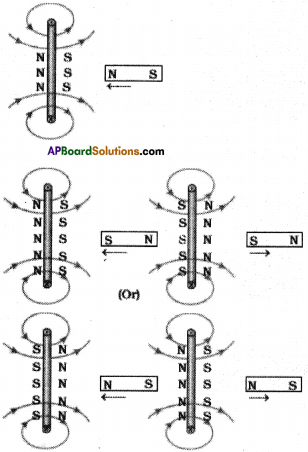Question 3.
What is the use of slip – ring in AC motor? (TS June 2018)
Uses of slip rings :
Slip rings are used to change the direction of current in the coil continuously.

Question 4.
Draw the direction of magnetic lines force, assuming that the current is flowing into the page. (AP SCERT: 2019-20 )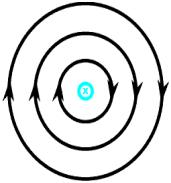Question 5.
What happens when a current carrying coil is placed in a uniform magnetic field? (TS June2019)
The rectangular coil comes into rotation in clock – wise direction because of equal and opposite pair of forces acting on the two sides of the coil.

1. If the direction of the current in the coil unchanged it rotates half clock – wise and comes to half and rotates in anticlock – wise direction.
2. If the direction of the current in the coil changed after the first half rotation, the coil continuously rotates in a same direction.Question 6.
Write the name of the device that converts mechanical energy into electrical energy. (AP June 2019)
Generator.

Question 7.
Name some sources of direct current.

Question 8.
Which sources produce alternating current?
A.C generator, thermal power station, hydroelectric stations.

Question 9.
What is the role of split ring in an electric motor?
The split rings are used to change the direction of current flowing through the coil.

Question 10.
Write one method of inducing current in the coil.
By pushing or pulling a bar magnet into or away from the coil we can induce current. Name two safety measures commonly used in electric circuit, i) Fuse ii) Earthing

Question 11.
On what factors does the magnetic induction at the centre of the coil depend?
It depends on current, number of turns and radius of the coil.Question 12.
Which is more dangerous AC or DC?
AC is more dangerous.

Question 13.
State two serious hazards of electricity.

• If a person touches the live wire, he gets severe shock which may prove fatal.
• Short-circuiting can cause a spark which may lead to fire in a building.

Question 14.
Why is earthing of electrical appliances recommended?
To protect the user from any accidental electrical shock caused due to leakage of current.

Question 15.
Why is a spark produced at the place of short circuit? Why is the spark in white colour?
The resistance of circuit decreases, and a sudden flow of large current heats up the live wire and vapourises the metal. This causes spark. The metal of wire becomes very hot and naturally emits white light.

Question 16.
What is electromagnetic induction?
Mechanical energy can be converted into electrical energy by moving a magnet inside a coil.

Question 17.
What is Maxwell’s right hand screw rule?
The direction of current is the direction in which the tip of the screw advances and direction of ration of the screw gives the direction of magnetic lines of force.

Question 18.
What type of energy transformation takes place in electric generator?
Electrical energy from mechanical energy.

Question 19.
Where are the electromagnets used?
In electric generators and televisions.

Question 20.
What is electromagnet?
When current carrying conductor is wound over a magnetic material like soft iron it gets magnetized.

Question 21.
What are the different types of power stations?
Electrical energy is produced in different power stations from mechanical energy of water, meat energy, and nuclear energy.Question 22.
If the current in the coil is in anti-clockwise, then what would be the face of the coil?
It behayes as north pole.

Question 23.
If the current in the ceil is in clockwise, then what would be the face of the coil?
It behaves as south pole.

Question 24.
What is the frequency of the A.C. supplied in your house?
It is approximately 50 Hz.

Question 25.
What type of current is generated in electric power station?
Alternating current.

Question 26.
What is the shape of magnetic lines due to straight current carrying conductor?
They are concentric circles.

Question 27.
What is a transformer?
It is a device which increases or decreases the voltage.

Question 28.
State two ways by which speed or rotation of electric motor can be increased.

1. By increasing strength of the current.
2. By increasing number of turns in the coil.

Question 29.
What happens if an iron piece is dropped between two poles of strong magnet?
Eddy current is produced in it. These eddy currents oppose the motion of the piece of iron. So it falls as it is moving through a viscous liquid.

Question 30.
If a copper rod carries a direct current, then where will be the magnetic field in the conductor?
It will be both inside and outside the rod.

Question 31.
In what form is the energy in a current carrying coil stored?
It is stored in the form of magnetic field.

Question 32.
What is solenoid?
A solenoid is a long wire wound in a close packed helix.

Question 33.
What is the pattern of field lines inside a solenoid around when current carrying solenoid?
Parallel to each other.

Question 34.
List any two properties of magnetic field lines.

• Inside the magnet they start from south pole and end at north pole whereas outside the magnet they start at north pole and end at south pole.
• Two magnetic lines of force never intersect each other.

Question 35.
Why does the picture appear distorted when the bar magnet is brought close to the screen of a television?
Picture on a television screen is due to motion of the electrons reaching the screen. These electrons are affected by magnetic field of bar magnet.Question 36.
What is meant by electromagnetic induction?
Whenever there is continuous change of magnetic flux linked with a closed coil, current generated in the coil is called electromagnetic induction.

Question 37.
State the Lenz’s principle.
The induced current will appear in such a direction that it opposes the changes in the flux in the coil.

Question 38.
What is induced emf?
Change in magnetic flux produces emf in the circuit called induced emf.

Question 39.
What do you mean by magnetic effect of current?
Current carrying conductor produces a magnetic field around it. This is called magnetic effect of current.

Question 40.
What is the direction of magnetic field at the centre of the coil carrying current in (i) clockwise, (ii) anti-clockwise direction?
i) Along the axis of coil inwards.
ii) Along the axis of coil outwards.

Question 41.
Why does a current carrying freely suspended solenoid rest along a particular direction?
A current carrying solenoid behaves like a bar magnet.

Question 42.
What effect will there be on a magnetic compass when it is brought near a current carrying solenoid?
The needle of the compass will rest in the direction of magnetic field due to solenoid at that point.

Question 43.
How is magnetic field due to solenoid carrying current affected, if a soft iron bar is introduced inside the solenoid?
The magnetic field increases when iron bar is introduced inside the solenoid.Question 44.
What happens to magnetic field if we reverse the current direction?
The magnetic field also gets reversed.

Question 45.
How do magnetic field lines inside a current carrying solenoid appear?
They form along the axis and parallel to each other.

Question 46.
In which of the following cases does the electromagnetic induction occur?
i) A current is started in a wire held near a loop of wire.
ii) The current is stopped in a wire held near a loop of wire.
iii) A magnet is moved through a loop of wire.
iv) A loop of wire is held near a magnet.
In first three cases there is a change in magnetic flux. So electromagnetic induction occurs in first three cases.

Question 47.
Why must an induced current flow in such a direction so as to oppose the change producing it?
So that the mechanical energy spent in producing the change, is transformed into the electrical energy in form of induced current.

Question 48.
What is the maximum force acting on the current (i) carrying conductor of length (l) in the presence of magnetic field (B)?
F = Bil

Question 49.
A charged particle q is moving with a speed v perpendicular to the magnetic field of induction B?
Find the equation of time period of the particle.
$$\mathrm{T}=\frac{2 \pi \mathrm{m}}{\mathrm{Bq}}$$

Question 50.
Name two safety measures commonly used in electric circuit.
a) Fuse
b) Earthing

Question 51.
On what factors the magnetic induction at the centre of coil depends?
a) Number of turns

Question 52.
Write the formula for magnetic flux passing through an Area A with an angle θ.
Flux ΦV= BA cos θQuestion 53.
Write the Lenz’s law.
The induced current will appear in such a direction that it opposes the changes in the flux in the coil.

Question 54.
What is the difference between AC and DC generator?
In AC generator, ends of coil are connected to two slip rings.
In DC generator ends of coil are connected to two half split rings.

Question 55.
What are the uses of electromagnet?
It is used in electric bells, electric motors, telephone diaphragms, etc.

Question 56.
What is the principle of Electric motor?
When a rectangular coil is placed in magnetic field and current is passed through it, two equal and opposite forces act on the coil which rotates it continuously.

Question 57.
What factors are influence the speed of rotation of the motor?

1. Strength of current
2. Number of turns
3. Area of the coil
4. Strength of magnetic field

Question 58.
Which two physical quantities are interrelated in Oerstead experiment?
Electricity and magnetism are interrelated.Question 59.
Which property of proton can change while it moves freely in a magnetic field?
When a proton (the charge) moves in a magnetic field, then magnetic force is acting on proton. So its momentum changes.

Question 60.
Which type of conductors are producing magnetic field?

1. Long straight current carrying conductor.
2. Circular loop.
3. Solenoid.

Question 61.
How much force acting on a neutron particle is moving with velocity V in a mag¬netic field with induction B?
Zero, because neutron is charge less particle.

Question 62.
What are the instruments used in A.C Generator?
Rectangular coil, brushes, slip rings, and magnetic poles.

Question 63.
What are the ways to produce the induced current in a coil?
When a magnet is moved towards or away from coil or there is a relative motion between coil and magnet a current is induced in the coil.

Question 64.
At the time of short circuit, what happens to the current?
At the time of short circuit, the current in the circuit increases heavily becomes the resistance of the conductor becomes almost zero.

Question 65.
A wire with green insulation is usually the line wire of an electric supply. Is it true?
It is false, the wire with green insulation is the earth wire, not the line wire.

Question 66.
Two circular coils A and B are placed close to each other. If the current in the coil A is changed, will some current be induced in the coil B? Give reason.
Yes. current will be induced in coil ‘B’, because flux linked the coil ‘B’ changes with respect to time.

Question 67.
Name two safety measures commonly used in electric circuits and appliances.
Electric fuse and Miniature Circuit Breaker (MCB).

Question 68.
An attemating current has frequency of 50Hz. How many times does it change its direction in one second?
Frequency of AC = 50 Hz ⇒ 50 cycles in one sec. So it reverses its direction 100 times in one second.

Question 69.
Under what orientation, the induced current produced in moving conductor in a magnetic field can be maximum?
The current induced in a conductor is maximum when direction of motion of conductor is at right angle to the magnetic field.Question 70.
How could you make the coil rotate continuously in motor?
The direction of current through the coil is reversed every half rotation, the coil will rotate continuously and the same direction.

Question 71.
What is the formula for induced cmf when change the magnetic flux?
Induced EMF = $$-\frac{\Delta \phi}{\Delta t}$$

Question 72.
The magnetic flux is varying with time. Which cases E.M.F is induced?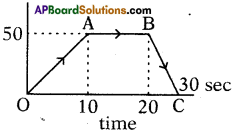In OA and BC cases, E.M.F is induced.

Question 73.
In above problem, how much EMF is induced in BC curve?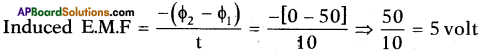Question 74.
What is the equation of motional E.M.F?
Motional E.M.F = Blv;
where B = magnetic induction,
l = length of rod,
v = velocity of rod.

Question 75.
When a magnet and a coil are moving same direction with same speed. Then induced E.M.F in coil is zero. Why?
E.M.F = $$\frac{-\Delta \phi}{\Delta \mathrm{t}}$$, but both moving same direction, so change in flux linked with coil is zero i.e., ∆Φ = 0

Question 76.
What is shape of conductor is drawn when current is passing through conductor?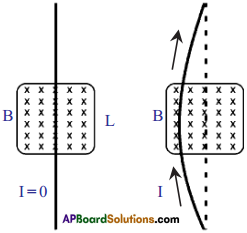Question 77.
Draw the symbols of North and South pole when depends on current.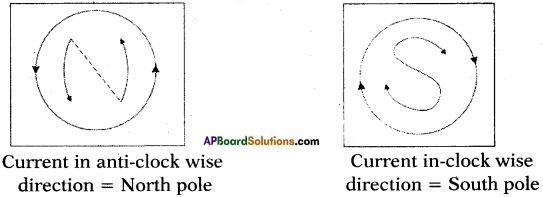Question 78.
The magnetic field in a given region is uniform. Draw a diagram to represent it.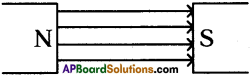Question 79.
Draw the diagram of AC and DC current varying with time.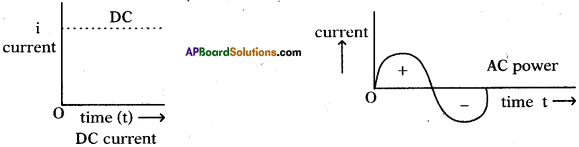Question 80.
Where are electric motors used?
Electric fans, water-pumps, coolers.

Question 81.
Mention two uses of solenoid.
It is used in electric bells, fans, and motors.

Question 82.
Mention applications of electromagnetic induction.
It is used in devices which convert mechanical energy into electrical energy.Question 83.
What are the advantages of an electromagnet over permanent magnet?

1. An electromagnet can produce a strong magnetic field.
2. The strength of electromagnet can be changed.
3. The polarity of electromagnet can be changed.

### 10th Class Physics 12th Lesson Electromagnetism 2 Marks Important Questions and Answers

Question 1.
Anand appreciated the law behind the making of ‘generator’. Name the law and state it. (AP June 2017)
1) The law behind the making of ‘generator’ is Faraday’s law.

“Whenever there is a continuous change of magnetic flux linked with a closed coil, a current is generated in the coil”.

Question 2.
Explain Oersted experiment to show that Electricity and Magnetism were related phenomena. (AP June 2018)

• Place a compass needle underneath a wire and then turn on electric current.
• Immediately the needle of compass shows the deflection. By this we can conclude that electricity and magnetism are related phenomena.

Question 3.
With the help of the given figure, the teacher explained that magnetic field lines are closed lines and not open lines. Write the questions which you will ask to rest whether the given statement is right or wrong. (TS June 2015)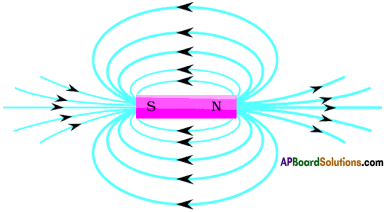• Are there any magnetic field lines inside the magnet?
• If magnetic field lines are there inside the magnet, what is the direction of field lines inside the magnet?
• What is the direction of field lines outside the magnet?

Question 4.
State Right-hand rule with a labelled diagram. (TS March 2015)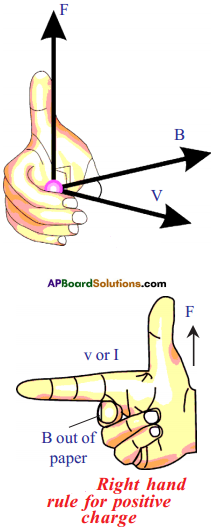(OR)

• Right hand rule indicates the direction of magnetic force acting on a moving charge.
• It is used when velocity and field are perpendicular to each other. If the fore finger points towards the direction of velocity of charge or current (I), middle finger points to the direction of field (B), then thumb gives the direction of force (F).

Question 5.
A coil of insulated Copper wire is connected to a Galvanometer. (TS March 2015)
What happens, if a bar magnet is ………….
1) pushed into the coil?
2) withdrawn from inside the coil?
3) held stationary inside the coil?

1. If a bar magnet is pushed into the coil, then the needle in Galvanometer gets deflected. Because current is generated in the coil.
2. If a bar magnet is withdrawn from inside the coil, then the needle in Galvanometer gets deflected. Because current is generated in the coil.
3. If a bar magnet is held stationary inside the coil, then the needle in Galvanometer does not get deflected. Because current is not generated in the coil.

Question 6.
Compare the magnetic field lines of force formed around a current carrying solenoid with the magnetic field lines of force of a bar magnet.

 Magnetic field lines of a bar magnet Magnetic field lines of a solenoid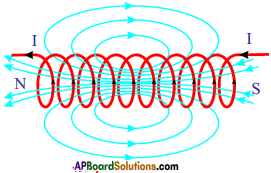2) The direction of the field lines of the outside the magnet is from north to south pole. 2) The direction of the field lines of the outside the solenoid is from north to south pole. 3) The direction of the field lines of the inside of the magnet field looks like south to north pole. 3) The direction of the field lines of the inside of the solenoid is from south to north pole. 4) These lines are closed loops. 4) These lines are also closed loops. 5) We cannot find the field lines inside the bar magnet. 5) We can find the field lines inside the solenoid. 6) These field lines are same as field lines formed by a solenoid. 6) These field lines are also same as field lines formed by a bar magnet. 7) More field lines are found at poles. 7) More field lines are found at poles.

Question 7.
Which energy we get from an electric motor? Write two daily life applications of the electric motor. (TS June 2017)

• We get mechanical energy from electric motor.
• In our daily life we use motor in
i) Mixies
ii) Grinders
iii) Water Pumps
iv) Fans / Coolers, etc.Question 8.
List out the material required for Oersted experiment and mention the precautions to be taken in the experiment. (TS March 2019)
Materials required for Oersted experiment are :

1. Thermocol sheet.
2. Wooden sticks.
3. Copper wire of 24 gauge.
4. Battery.
5. Key.
6. Magnetic compass.

Precautions to be taken are :

1. Copper Wire is made through the slits of the wooden sticks tightly.
2. Arrange/complete the circuit correctly.

Question 9.
What happens when magnetic flux passing through a coil changes continuously? Where does this process is used? (TS June 2019)
1) When there is a continuous change of magnetic flux passing through a coil a current is generated in the coil.

2) This process is used in

1. Induction stoves
2. Security checking entrance/exit doors.
3. ATM cards scanners.

Question 10.
Distinguish between AC and DC.

 AC DC 1) AC means alternate current. 1) DC means direct current. 2) The current direction changes 2) The current direction does not always. change. It is always a single direction. 3) The magnitude of current changes from minimum to maximum. 3) Always it has maximum value.

Question 11.
Distinguish between AC motor and DC motor.

 AC motor DC motor 1) It works with alternate current. 1) It works with direct current. 2) It does not require change in current direction. 2) It requires change in current direction which is provided by split rings which act as commutator.

Question 12.
Distinguish between AC generator and DC generator.

 AC generator DC generator 1) It generates alternate current. 1) It generates direct current. 2) It has two slip rings. 2) It has two half slip rings.

Question 13.
What is Faraday’s law of electromagnetic induction? Write its expression.
(OR)
State the Faraday’s law of electromagnetic induction. Write the equation of this law.
The induced EMF generated in a closed loop is equal to the rate of change of magnetic flux passing through it.
Induced EMF = Change in flux / time
ε = ∆Φ / ∆t

Let Φ0 be flux linked with single turn. If there are N turns of the coil, the flux linked with the coil is NΦ0.
∴ ε = NAΦ0 / ∆t

Question 14.
State the right-hand thumb rule. How does the rule help us?
When you curl your right hand fingers in the direction of current thumb gives the direction of magnetic field.

It is useful to find the magnetic field direction as well as current direction.Question 15.
A flat rectangular coil is rotated between the pole pieces of a horseshoe magnet. In which position of coil with respect to magnetic field, will the emf (i) be maximum, (ii) be zero, (iii) change its direction.
i) The emf is maximum when the plane of coil is parallel to the magnetic field.
ii) The emf is zero when the plane of coil is normal to the magnetic field.
iii) The emf will change its direction when the plane of coil passes from the position normal to the magnetic field.

Question 16.
State two factors on which the magnitude of induced emf depend?
The magnitude of induced emf depends on the following two factors.

1. The change in the magnetic flux.
2. The time in which the magnetic flux changes i.e., the rate of change of magnetic flux.

More the change in magnetic flux, more is the emf induced. Further if more rapid the magnetic flux changes, more is the emf induced.

Question 17.
How do you increase the magnetic field of solenoid?
The magnetic field of solenoid can be increased by the following two ways.

1. by increasing the number of turns of winding in the solenoid.
2. by increasing the current through the solenoid.

Question 18.
State the function of split ring in a DC motor.

• The split ring acts as a commutator in a DC motor.
• With the split ring, the direction of current through the coil is reversed after every half rotation of coil.
• Thus the direction of couple rotating the coil remains unchanged and the coil continues to rotate in the same direction.

Question 19.
A DC motor is rotating in a clockwise direction. How can the direction of rotation be reversed?
The direction of rotation of motor can be reversed by interchanging the connections at the terminals of the battery joined to the brushes of the motor.Question 20.
State the effect of inserting a soft iron core within the coil of DC motor.

• On inserting a soft iron core within the coil of a DC motor, the speed of rotation of coil increases.
• The reason is that the strength of magnetic field between the pole pieces of magnet increases due to which the deflecting couple on coil increases.

Question 21.
State condition when magnitude of force on a current-carrying conductor placed in a magnetic field is (a) zero, (b) maximum.
a) When current in conductor is in direction of magnetic field,
b) When current in conductor is normal to the magnetic field.

Question 22.
A flat coil ABCD Is freely suspended between the pole pieces of a U – shaped permanent magnet with the plane of coil parallel to the magnetic field.
a) What happens when current is passed in the coil?
b) When will the coil come to rest?
c) Name the instrument which makes use of the principle stated above.
a) The coil will experience a torque due to which it will rotate.
b) The coil will come to rest when its plane becomes normal to the magnetic field.
c) DC motor.

Question 23.
Why is it more difficult to move a magnet towards a coil which has a large number of turns?

1. Emf induced in the coil becomes more when the number of turns in the coil are made large.
2. So it is difficult to move a magnet towards a coil which has a large number of turns.

Question 24.
A coil ABCD mounted on an axle is placed between the poles N and S of permanent magnet as shown in figure.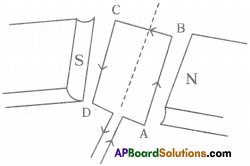a) In which direction will the coil begin to rotate when the current is passed through the coil in direction ABCD by connecting a battery at the ends A and D of the coil.
b) Why is commutator necessary for continuous rotation of the coil?
a) Anti-clockwise direction.
b) Commutator is needed to change the direction of current in the coil after each half rotation of coil.

Question 25.
Draw the magnetic field lines in uniform magnetic field.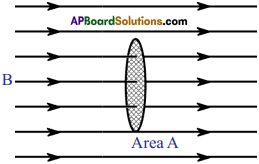Question 26.
Draw a labelled diagram to make an electromagnet from soft iron bar AB. Mark the polarity at its ends. What precaution would you observe?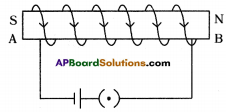The labelled diagram is shown in figure. The polarity at the end A where the current is clockwise, is south (S), while at the end B where the current is anti-clockwise is north (N).
Precaution :
The source of current must be the DC source.

Question 27.
State the principle of an electric motor. Name some appliances in which the Electric motor is used.
Current carrying coil rotates when it is kept in a uniform magnetic field. It is the working principle of electric motor.
Appliances containing electric motor are :

1. Fans,
2. Mixies,
3. Grinders,
4. Machines, etc.

### 10th Class Physics 12th Lesson Electromagnetism 4 Marks Important Questions and Answers

Question 1.
How can you verify with experiment “The magnetic field lines are closed loops”? (AP March 2017)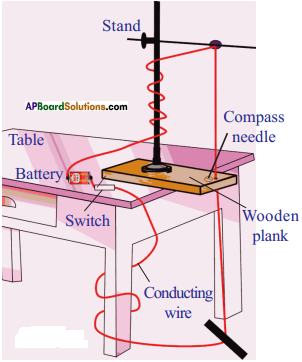• Place a retort stand on the plank as shown in the figure.
• Pass a copper wire through hole of the plank and rubber knob of the retort stand in such a way that the wire be arranged in a vertical position and not touch the stand.
• Connect the two ends of the wire to a battery via switch. Allow the current flows through wire.
• By sprinkling the iron fillings around the wire, we can observe the magnetic field lines are in circular shape.

Conclusion :
Hence it is proved that “Magnetic field lines are closed loops”.

Question 2.
Name the device that converts electrical energy into mechanical energy. Draw its diagram and label the parts. (AP March 2018)
1) The device that converts electrical energy into mechanical energy is motor.
2) Diagram of motor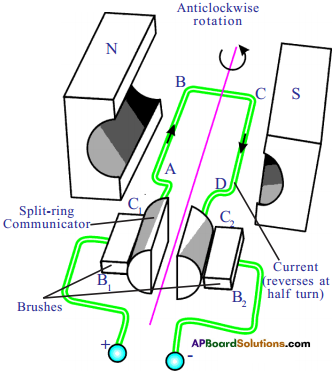Question 3.
List out the materials required for the Oersted experiment of electromagnetism. Write the procedure of the experiment. What do you understand by this experiment? (TS March 2016)
List of material required for Oersted experiment:
A thermocol sheet, two small sticks, insulated copper wires, 9 V battery, switch, magnetic compass.

Procedure :

1. Take a thermocol sheet and fix two thin wooden sticks of height 1 cm which have small slit at the top of their ends.
2. Arrange a copper wire so that it passes through these slits and makes a circuit.
3. The circuit consists of a 9 V batten’, key, and copper wires connected in series.
4. Now keep a magnetic compass below the wire.
5. Now switch on the circuit and observe the compass needle.
6. Change the directions of current and observe the compass needle.

Observation :

1. When current is passed through circuit, we observe deflection of compass needle in one direction.
2. When the direction of current is changed, the compass needle deflects in another direction.
3. This shows that a current carrying conductor possesses magnetic field around it.Question 4.
Write the experimental procedure and observations of the experiment that is to be performed to observe the magnetic field formed due to solenoid. (TS June 2017)

• Fix a white paper on a wooden plank.
• Make two holes to the plank at appropriate distance.
• Make some more holes parallel to these two holes.
• Insert a copper wire through these holes. It looks as a coil.
• Connect the two ends of the coil to a battery and a switch in series.
• When swich is on current flows through the wire.
• Sprinkle some iron filings around the coil and tap the plank.

Observation :
An orderly pattern of iron filing is seen on the paper. These are magnetic field lines. The magnetic field lines set up by solenoid resemble those that of a bar magnet.

Question 5.
Why the current-carrying straight wire which is kept in a uniform magnetic field, perpendicularly to the direction of the field bends aside? Explain this process with a diagram showing the direction of forces acting on the wire. (TS March 2017)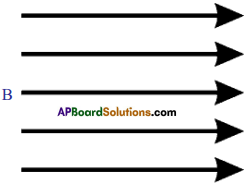Uniform magnetic field (due to horse shoe magnet)Magnetic field due to current carrying straight wire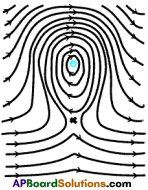Net field formed due to the above two fields

Explanation :
The net field in upper part is strong and in lower part it is weak. Hence a non-uniform field is created around wire. Therefore the wire tries to move to the weaker field region.

Question 6.
List out the apparatus and experimental procedure for the experiment to observe a current-carrying wire experiences a magnetic force when it is kept in uniform magnetic field. (TS June 2018)
Required apparatus :
i) Horseshoe magnet,
ii) Conducting wire,
iii) Battery, switch

Experimental procedure :
1) Arrange the circuit :
Take a wooden plank and arrange two wooden sticks with slits and arrange a conductor through the sticks and make a circuits with switch, battery.

2) Put horse shoe magnet:
Arrange the horse shoe magnet on the conductor in such a way that the conductor should be in between the two poles of the magnet.

3) Deflections in conductor :
Allow the current to pass through the circuit. We can find that conductor deflects up wards or down wards.

4)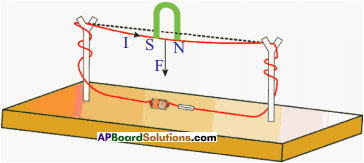Question 7.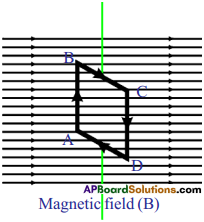Answer the following questions by observing above diagram. (TS March 2018)
1. Which device function of working does the above figure gives?
Motor

2. What is the angle made by AB and CD with magnetic field?
90°

3. What are the directions of magnetic forces on sides AB and CD?
By applying right hand rule to get the directions of magnetic force. At ‘AB’, the magnetic force acts inward perpendicular to field of the magnet and on ‘CD’, it acts outwards.

4. What is the net force acting on the rectangular coil?
The net force on ‘AB’ is equal and opposite to the force on ‘CD’ due to external magnetic field because they carry equal currents in the opposite direction. Sum of these forces is zero. Similarly, the sum of the forces on sides ‘BC’ and ‘DA’ is also zero.

So, net force on the rectangular coil is zero.

Question 8.
Explain the working process of induction stove. (TS March 2019)

• An induction stove works on the principle of electromagnetic induction.
• A metal coil is kept just beneath the cooling surface.
• It carries alternating current (AC) so that AC produce an alternating magnetic field.
• When you keep a metal pan with water on it, the varying magnetic field beneath it crosses the bottom surface of the pan, and EMF is induced in it.
• Induced EMF produces induced current in the metal pan.
• The pan has a finite resistance.
• The flow of induced current produces heat in it.
• That heat is conducted to the water. In this way, induction stove works and water will be heated.

Question 9.
Which device is used to convert mechanical energy into electrical energy? Draw a neat diagram and label the parts of this device. (TS March 2019)
1) Dynamo is used to convert mechanical energy into electrical energy. They are also called Generators.

2) AC Generator (or) DC Generator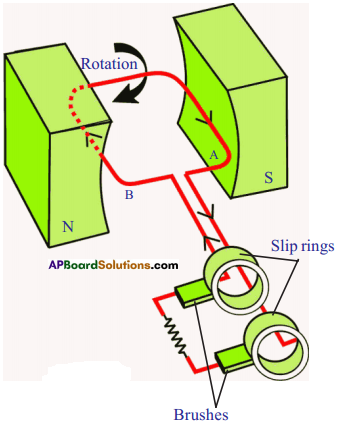AC Generator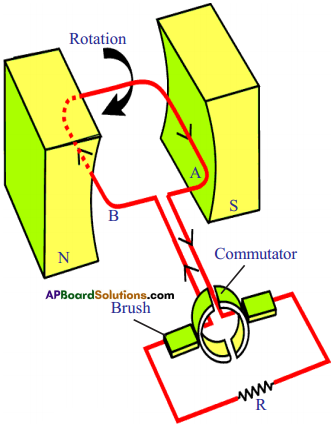DC Generator

Question 10.
A coil is hung as shown in the figure. A bar magnet with north pole facing the coil is moved perpendicularly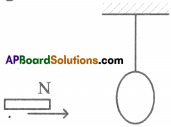a) How does the magnetic flux passing through the coil change?
b) State the direction of the flow of the current induced in the coil, keeping the direction of bar magnet in view.
c) Draw the diagrams showing the magnetic field formed due to bar magnet at the surface of the coil and the magnetic field formed due to induced current.
d) Explain the reason for induced current.
a) A bar magnet with north pole facing the coil is moved perpendicularly, the magnetic flex increases when passing through the coil.
b) The direction of the flow of the. current induced in the coil, keeping the direction of bar magnet is anti-clockwise due to north pole. KT
c) Φ = 0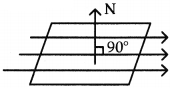Plane of coil is parallel to ‘B’.
d) Electromagnetic induction is the reason for induced current.

Question 11.
Conductor of length T moves perpendicular to its length with the speed V. Length of the conductor is perpendicular to the magnetic field of the conductor. Let us assume that electrons could move freely in the conductor and the charge of an electron is ‘e’.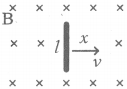a) What is the magnetic force acting on electron in the conductor?
b) In which direction does the above force act?
c) What effect does this force have on motion of electrons?
a) Magnetic field acting on the electron inside the conductor is = Fm = e ($$\bar{V} \times \bar{B}$$) = BeV
This field acts from P to Q.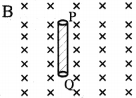b) Consider in the field P and Q are ends of a conductor. ‘Q’ will act as negative end and ‘P’ will act as positive end then flow passes from P to Q means downwards.

c) The force on electrons shows an effect creates a potential difference at the ends of the rods.
∴ BeV = eE ⇒ E = BY

Question 12.
A charged particle q is moving with a speed V perpendicular to the magnetic field induction B. Find the radius of the path and time period of the particle.

1. Let us assume that the field is directed into the page.
2. Then the force experienced by the particle F = qvB.
3. We know that this force is always directed perpendicular to velocity.
4. Hence the particle moves along a circular path and the magnetic force on a charged particle acts like a centripetal force.
5. Let r be the radius of the circular path.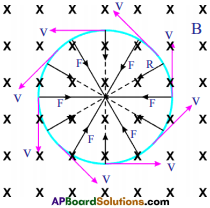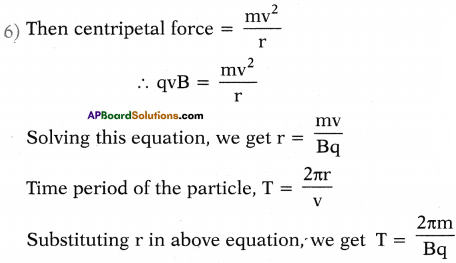Question 13.
Explain different ways to induced current in a coil.

• Moving a north pole of a magnet into a coil.
• Withdrawing north pole from a coil.
• Moving a south pole of magnet into a coil.
• Withdrawing a south pole of a magnet from a coil.
• Moving a coil towards a magnet.Question 14.
What are the similarities between a current-carrying solenoid and a bar magnet?
1) The magnetic field lines due to current carrying solenoid are identical to those of bar magnet. Thus a current-carrying solenoid behaves just like a bar magnet with fixed polarities at the ends. The end at which the direction of current is clockwise behaves like a south pole and the end at which current is anti-clockwise behaves like a north pole.

2) A current-carrying solenoid when suspended freely, will set itself in the north south direction exactly in the same manner as a bar magnet does.

3) A current-carrying solenoid also acquires the- attractive property of magnet. If iron filings are brought near the solenoid, it attracts them when current flows through the solenoid.

Question 15.
What are the dissimilarities between a current-carrying solenoid and a bar magnet?

• The magnetic field strength due to solenoid can be altered by altering current in it, while the magnetic field strength of a bar magnet cannot be changed.
• The direction of magnetic field due to solenoid can be reversed by reversing the direction of current in it, but the direction of magnetic field of the bar magnet cannot be changed.

Question 16.
Compare electromagnet with a permanent magnet.

 Electromagnet Permanent Magnet 1) It is made of soft iron. 1) It is made of steel. 2) It produces the magnetic field so long as current flows in its coils. 2) It produces permanent magnetic field. 3) The magnetic field strength can be changed. 3) The magnetic field strength cannot be changed. 4) The electromagnet can be made as strong as needed. 4) The permanent magnets are not so strong. 5) The polarity of an electromagnet can be reversed. 5) The polarity of permanent magnet cannot be reversed. 6) It can be easily de-magnetised by switching off the current. 6) It cannot be easily de-magnetised.

Question 17.
What are the characteristics of magnetic field lines due to current in a loop (or circular coil)?

• The magnetic lines are nearly circular in the vicinity of coil.
• Within the space enclosed by the wire the magnetic field lines are in the same direction.
• Near the centre of loop, the magnetic field lines are nearly parallel and the magnetic field may be assumed to be uniform in a small space near the centre.
• At the centre, the magnetic lines are along the axis of the loop and at right angles to the plane of the loop.
• The magnetic field lines become denser if the strength of current in the loop is increased and there are more number of turns in the loop.

Question 18.
A straight conductor passes vertically through a cardboard having some iron filings sprinkled on it.
a) Show the setting of iron filings when current is passed in the downward direction and then the cardboard is gently tapped. Draw arrows to represent the direction of magnetic field lines.
b) What changes occur if
i) current is increased ?
ii) the single conductor is replaced by several parallel conductors each carrying same current flowing in the same direction?
c) Name the law used by you to find the direction of magnetic field lines.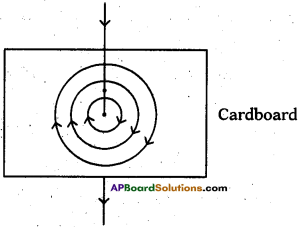a) The figure shoy/s, the pattern in which iron filings will set themselves. The arrows show the direction of magnetic field lines.
b) i) The arrangement of iron filings remains unchanged, but they become denser and Cardboar get arranged up to a larger distance from the conductor when the strength of current is increased and it is effective up to a larger distance from the conductor.
ii) The magnetic field at a point due to each conductor will be in same direction, so they will be added up. Thus the magnetic field strength is increased and it is effective up to a large distance so the magnetic field lines come closer and iron filings get arranged up to a larger distance.
c) Right hand thumb rule.

Question 19.
In figure A and B represent two straight wires carrying equal currents in a direction normal to the plane of paper inwards.
a) Sketch separately the magnetic field lines produced by each current.
b) Give a reason why the magnetic field at K (mid point of the line joining A and B) will be zero.
c) What will be the effect on the magnetic field at the point K if the current in wire B is reversed?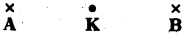a) Figure shows the sketch of magnetic field A and B.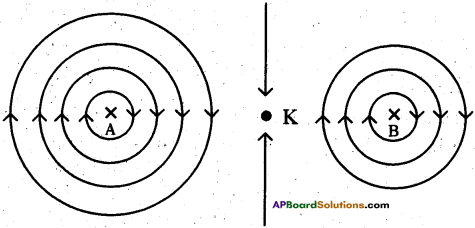b) The point K is equidistant from the wires A and B, and the wires A and B carry equal currents. So the magnetic fields at K due to wires A and B are equal in magnitude, but opposite in direction. Due to the wire A, it is downwards in the plane of paper, while due to the wire B, it is upwards in the plane of paper. So the net magnetic field at the point K is zero as the two fields cancel each other.
c) On reversing the direction of current in the wire B, the direction of magnetic field due to current is reversed at the point K, i.e. it becomes downwards in the plane of paper.

Question 20.
The diagram given below shows two coils X and Y. The coil X is connected to a battery S and a key K. The coil Y is connected to a galvanometer G.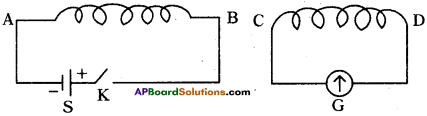When the key K is closed state the polarity.
i) At the end B of the coil X.
ii) At the end C of the coil Y.
iii) At the end C of the coil Y if the coil Y is (a) moved towards the coil X, (b) moved away from the coil X.
i) On closing the key K, the current at the end B of the coil X is anti-clockwise, therefore at this end there is a north pole.

ii) While closing the key, polarity at the end C of the coil Y will be north. But there will be no polarity at the end C of the coil Y when the current becomes steady in the coil X

iii) a) With the key K closed, while the coil Y is moved towards the coil X, the polarity at the end C of the coil Y is north.
b) With the key K closed, while the coil Y is moved away from the coil X, the polarity at the end C of the coil Y is south.

Question 21.
How do you increase the speed of rotation of coil in a DC motor?
The speed of rotation of coil can be increased by

1. Increasing the strength of current.
2. Increasing the number of turns in coil.
3. Increasing the strength of magnetic field. To increase the strength of magnetic field a soft iron core can be inserted within the coil.Question 22.
Explain Lenz’s law with an activity.
Lenz law :
The induced current will appear in such a direction that it opposes the change in the flux in the coil.

Explanation :

1. We know that when a bar magnet is pushed towards a coil with its north pole facing the coil an induced current is set up in the coil.
2. Let the direction be clockwise then current carrying loop behaves like a magnet with its south pole facing the north pole of bar magnet.
3. In such a case, the bar magnet attracts the coil. Then it gains kinetic energy. This is contradictory to conservation of energy.
4. Hence our assumption is wrong. So correct induced current direction is anti-clockwise.
5. Let us see a case where the bar magnet is pulled away from the coil with the north pole facing the coil. In such case, the coil opposes the motion of bar magnet to balance the conversion of mechanical energy into electric energy.
6. It happens only when the north pole of the magnet faces the south pole of the coil.
7. So, the direction of induced current in the coil must be in anti-clockwise direction.
8. In simple terms, when flux increases through coil, the coil opposes the increase in the flux and when flux decreases through coil, it opposes the decrease in the flux. This is Lenz law.

Question 23.
What are the uses of electromagnet?
Electromagnets are mainly used for the following purposes.

1. For lifting and transporting the large masses of scrap, girders, plates, etc. especially to the places where it is not convenient to take the help of human labour.